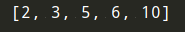# 《算法图解》学习笔记（二）：选择排序（附代码）

## 一、内存的工作原理fe0ffeeb是一个内存单元的地址，就相当于是机器给你的那个小票。

## 二、数组和链表• 你额外请求的位置可能根本用不上，这将浪费内存。你没有使用，别人也用不了。
• 待办事项超过10个后，你还得转移。

### 1）链表### 2）数组### 3）术语### 4）在中间插入### 5）删除PS：

• 不同：链表是 链式 的存储结构；数组是 顺序 的存储结构。
• 相同：两种结构均可实现数据的顺序存储，构造出来的模型呈 线性结构

## 三、选择排序• 电话簿中的人名
• 旅行日期
• 电子邮件（从新到旧）

python版本代码如下：

#查找数组中的最小值
def findSmallest(arr):
#存储最小值
smallest = arr
#存储最小值的索引
smallest_index = 0
for i in range(1, len(arr)):
if arr[i] < smallest:
smallest_index = i
smallest = arr[i]
return smallest_index

#排序数组
def selectionSort(arr):
newArr = []
for i in range(len(arr)):
#查找数组中最小的元素并将其添加到新数组中
smallest = findSmallest(arr)
newArr.append(arr.pop(smallest))
return newArr

print(selectionSort([5, 3, 6, 2, 10]))c++版本代码如下：

#include <iostream>
#include <vector>

using std::cout;
using std::endl;

// Finds the smallest value in an array
template <typename T>
int find_smallest(const std::vector<T>& arr) {
// stores smallest value
T smallest = arr;
// stores index of the smallest value
int smallest_index = 0;

for (int i = 0; i < arr.size(); i++) {
if (arr[i] < smallest) {
smallest = arr[i];
smallest_index = i;
}
}

return smallest_index;
}

template <typename T>
std::vector<T> selection_sort(std::vector<T> arr) {
std::vector<T> sorted;

while(!arr.empty()) {
// find smallest element and add it to sorted array
int smallest_index = find_smallest(arr);
sorted.push_back(arr[smallest_index]);

// remove smallest element from non-sorted array
arr.erase(arr.begin() + smallest_index);
}

return sorted;
}

int main() {
std::vector<float> arr = {1.2, 1.0, 3, 0, -1, 0.5, 100, -99};
std::vector<float> sorted = selection_sort(arr);

cout << "Sorted array: ";
for (float num : sorted) {
cout << num << " ";
}
cout << endl;
}


## 四、总结

• 计算机内存犹如一大堆抽屉。
• 需要存储多个元素时，可使用数组或链表。
• 数组的元素都在一起。
• 链表的元素是分开的，其中每个元素都存储了下一个元素的地址。
• 数组的读取速度很快。
• 链表的插入和删除速度很快。
• 在同一个数组中，所有元素的类型都必须相同（都为int、double等）。

## 参考文章